• Slides: 17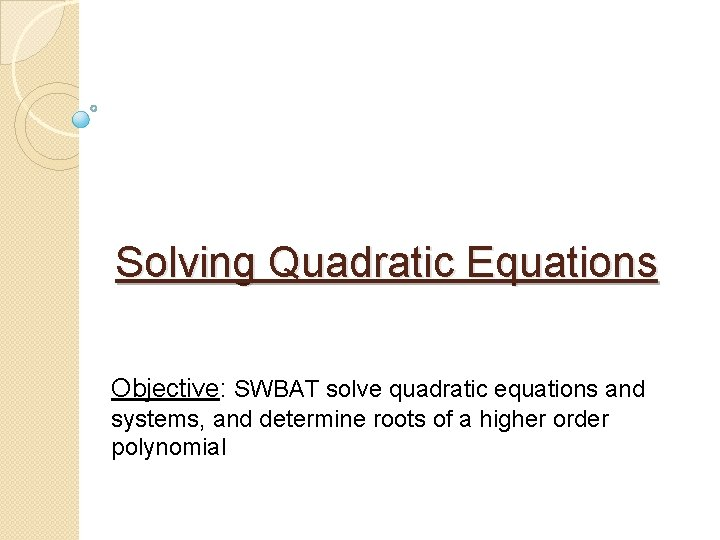Solving Quadratic Equations Objective: SWBAT solve quadratic equations and systems, and determine roots of a higher order polynomialWarm Up Factor the polynomial. 1. x 2 – 49 2. x 2 + 6 x + 5 3. 3 x 2 +10 x – 8 4. 3 x 2 -24 5. 8 x 2 + 38 x -10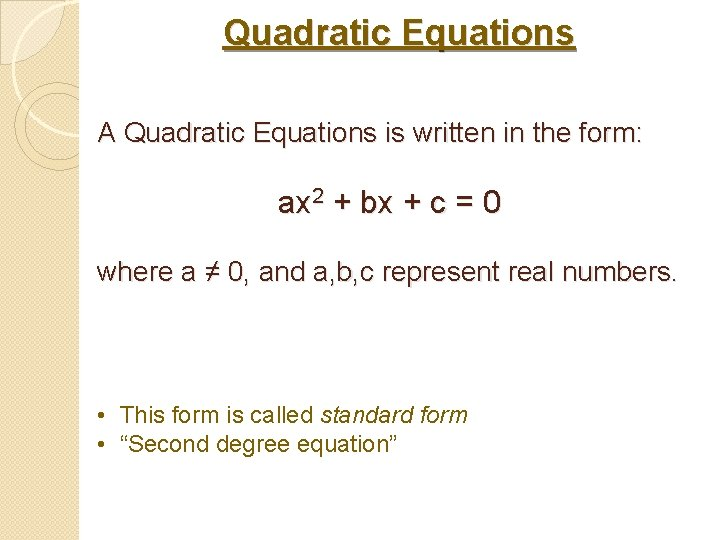Quadratic Equations A Quadratic Equations is written in the form: ax 2 + bx + c = 0 where a ≠ 0, and a, b, c represent real numbers. • This form is called standard form • “Second degree equation”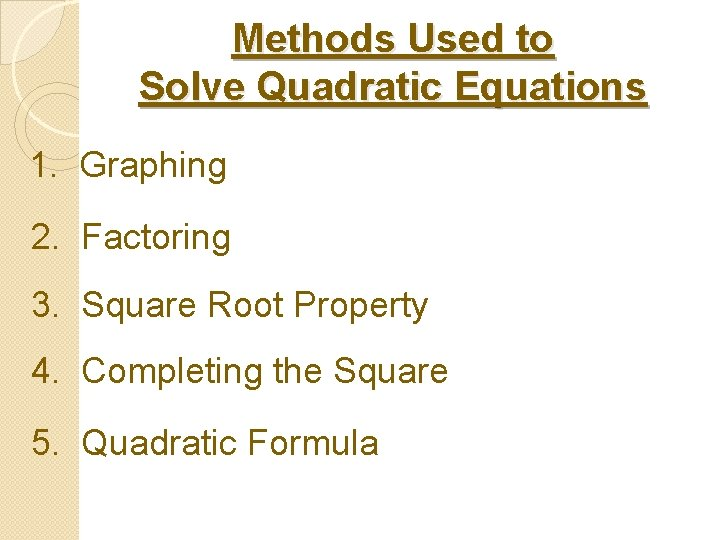Methods Used to Solve Quadratic Equations 1. Graphing 2. Factoring 3. Square Root Property 4. Completing the Square 5. Quadratic Formula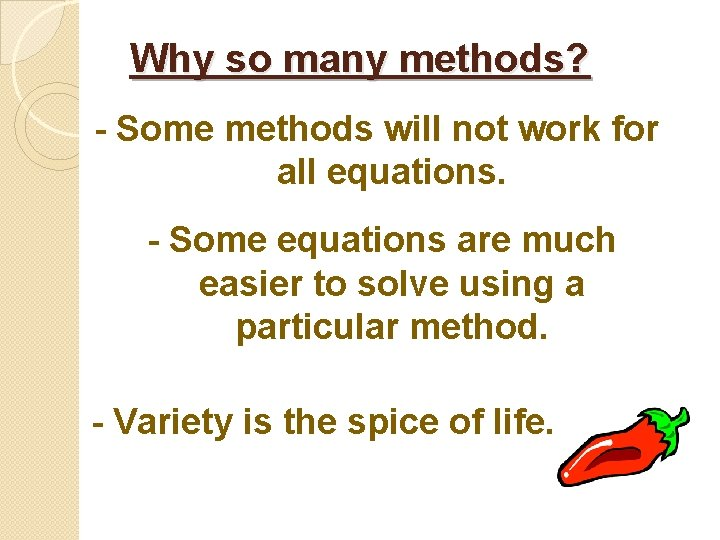Why so many methods? - Some methods will not work for all equations. - Some equations are much easier to solve using a particular method. - Variety is the spice of life.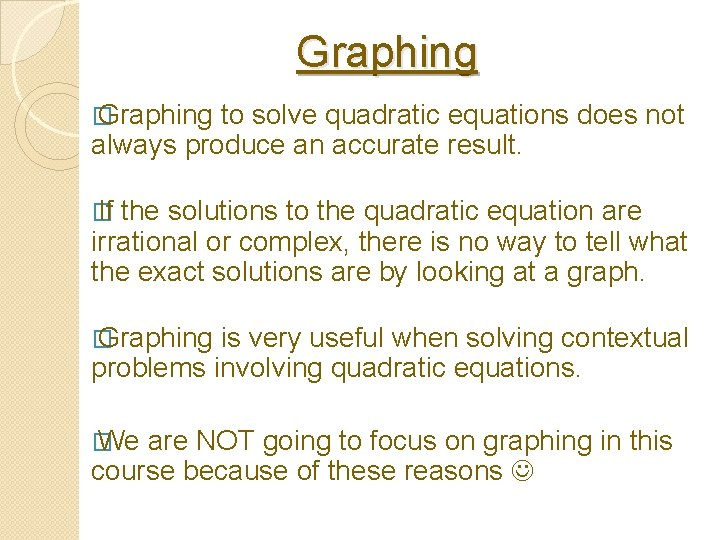Graphing � Graphing to solve quadratic equations does not always produce an accurate result. � If the solutions to the quadratic equation are irrational or complex, there is no way to tell what the exact solutions are by looking at a graph. � Graphing is very useful when solving contextual problems involving quadratic equations. � We are NOT going to focus on graphing in this course because of these reasons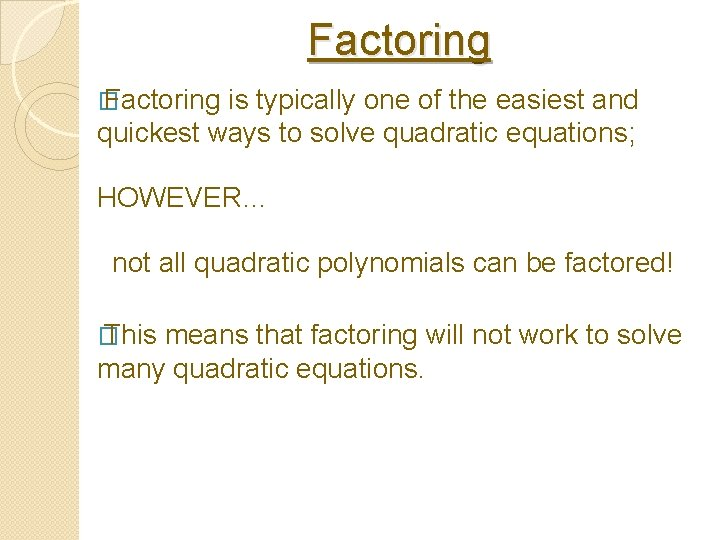Factoring � Factoring is typically one of the easiest and quickest ways to solve quadratic equations; HOWEVER… not all quadratic polynomials can be factored! � This means that factoring will not work to solve many quadratic equations.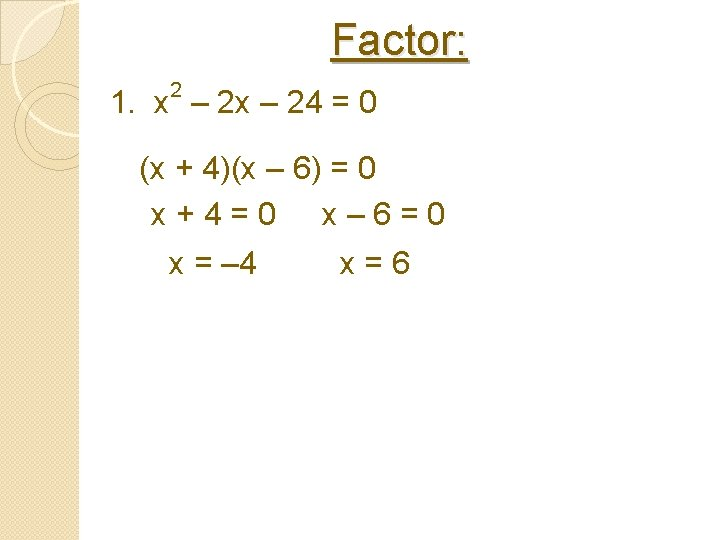Factor: 2 1. x – 24 = 0 (x + 4)(x – 6) = 0 x+4=0 x– 6=0 x = – 4 x=6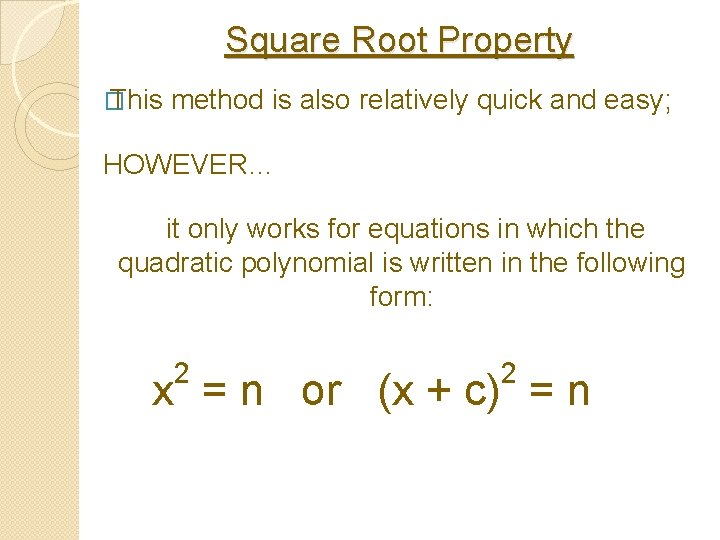Square Root Property � This method is also relatively quick and easy; HOWEVER… it only works for equations in which the quadratic polynomial is written in the following form: 2 2 x = n or (x + c) = n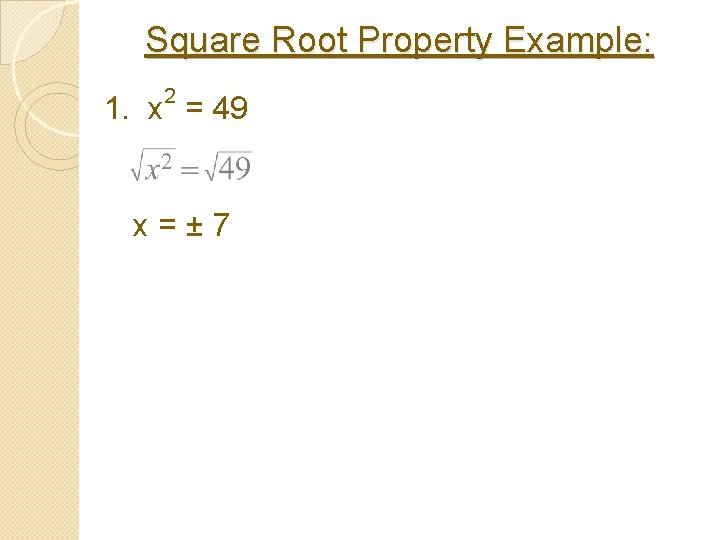Square Root Property Example: 2 1. x = 49 x=± 7Square Root Property Example: 2 2. (x + 3) = 25 x+3=± 5 x+3=5 x=2 x + 3 = – 5 x = – 8Completing the Square � This method will work to solve ALL quadratic equations! HOWEVER… � it is “messy” to solve quadratic equations by completing the square if a ≠ 1 and/or b is an odd number. Completing the square is a great choice for solving quadratic equations if a = 1 and b is an even number.Completing the Square Example: 2 x – 6 x + 13 = 0 2 x – 6 x + 9 = – 13 + 9 2 (x – 3) = – 4 x – 3 = ± 2 i x = 3 ± 2 iQuadratic Formula � This method will work to solve ALL quadratic equations!!! HOWEVER… for many equations it takes longer than some of the methods discussed earlier. YOU WILL BE HELD RESPONSIBLE FOR MEMORIZING THIS FORMULA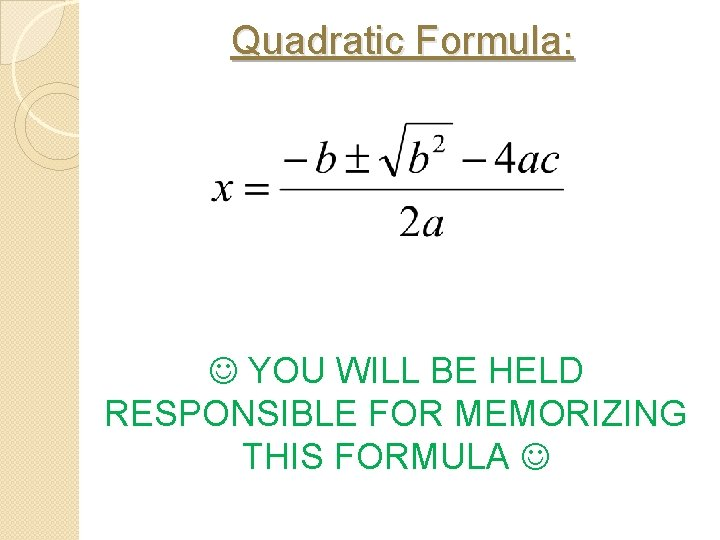Quadratic Formula: YOU WILL BE HELD RESPONSIBLE FOR MEMORIZING THIS FORMULAQuadratic Formula Example: 2 x – 8 x – 17 = 0 a=1 b = – 8 c = – 17Homework Page 441 # 34, 36, 40, 42, 48, 50